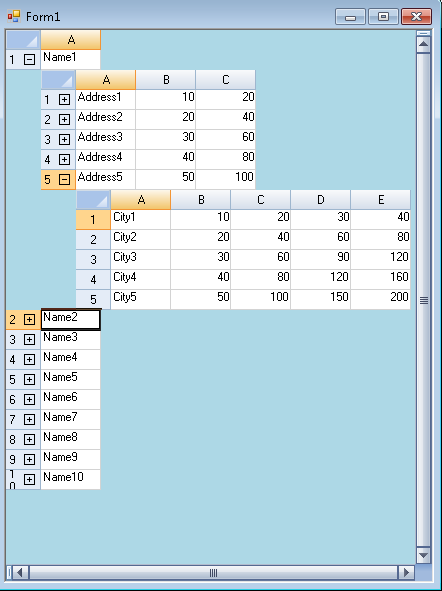﻿ Multiple Levels of Unbound Hierarchy in Spread# Multiple Levels of Unbound Hierarchy in Spread

Many a times users want to display related data in grids. The related data basically displays the data relations between different tables in a dataset. Spread lets you display such data with the help of bound hierarchy mode. Please refer to the following link that describes the concept in details: http://helpcentral.componentone.com/NetHelp/SpreadNet6/WF/spwin-databind-hierarchy.html But, sometimes the related data is not present in the form of a dataset with relations, rather the user manually defines the related data. Spread also lets you display the manually created hierarchy. You may refer to the following link that describes how you can implement one level of manual hierarchy : http://helpcentral.componentone.com/NetHelp/SpreadNet6/WF/spwin-hierarchy-manual.html However, there is a possibility that you might need to extend this manual hierarchy to multiple levels. This blog describes how you can define two levels of hierarchy. You can extend it further as per your specifications. This is how the final output would look like :The link above already describes the two methods that need to be overriden i.e. GetChildView(), FindChildView() and the property named ChildRelationCount. The two tricks that need to be followed to implement multiple levels are:

1. To set the parent for the child sheet. This is done by assigning the current sheet to the child sheet in the GetChildView() method. Each child sheet has a property named Parent that lets you define the relation.
2. The FindChildView method that is used to find the child view for a row is overriden in the class that represents the highest level of hierarchy. This method basically finds the child view for the expanded row. If no child view exists, it creates a new child view so that the remaining levels are created accordingly.

Here is the code snippet that creates two levels of hierarchy:

``````
{
public override int ChildRelationCount
{
get
{
return 1;
}
}
public override FarPoint.Win.Spread.SheetView GetChildView(int row, int relationIndex)
{
customSheet child = (customSheet)FindChildView(row, 0);
if ((child != null))>
return child;
child = new customSheet();
child.RowCount = 5;
child.ColumnCount = 3;
int r = 0;
for (r = 0; r &lt;= child.RowCount - 1; r++)
{
child.Cells[r, 0].Value = "Address" + (r + 1).ToString();
child.Cells[r, 1].Value = (r + 1) * 10;
child.Cells[r, 2].Value = (r + 1) * 20;
}
child.Parent = this;
child.SheetName = row.ToString();
return child;
}
public override FarPoint.Win.Spread.SheetView FindChildView(int row, int relationIndex)
{
string id = row.ToString();
foreach (customSheet CView in ChildViews)
{
if (CView.SheetName == id)
return CView;
}
return null;
}
}
{
public override int ChildRelationCount
{
get { return 1; }
}
public override FarPoint.Win.Spread.SheetView GetChildView(int row, int relationIndex)
{
customChild child = (customChild)FindChildView(row, relationIndex);
if ((child != null))
return child;
child = new customChild();
child.RowCount = 5;
child.ColumnCount = 5;
int r = 0;
for (r = 0; r &lt;= child.RowCount - 1; r++)
{
child.Cells[r, 0].Value = "City" + (r + 1).ToString();
child.Cells[r, 1].Value = (r + 1) * 10;
child.Cells[r, 2].Value = (r + 1) * 20;
child.Cells[r, 3].Value = (r + 1) * 30;
child.Cells[r, 4].Value = (r + 1) * 40;
}
child.Parent = this;
child.SheetName = row.ToString();
return child;
}
public override int ParentRowIndex
{
get { return Convert.ToInt32(SheetName);
}
}
{
public override int ParentRowIndex
{
get { return Convert.ToInt32(SheetName);
}
}

``````